# Differential Calculus & Functions Chapter Exam

Exam Instructions:

Choose your answers to the questions and click 'Next' to see the next set of questions. You can skip questions if you would like and come back to them later with the yellow "Go To First Skipped Question" button. When you have completed the practice exam, a green submit button will appear. Click it to see your results. Good luck!

### Page 1

#### Question 1 1. Decompose this composite function.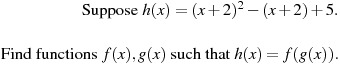#### Question 2 2. Decompose this composite function in multiple ways.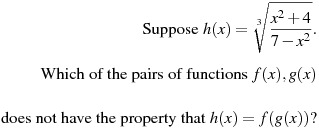#### Question 3 3. According to the mean value theorem, at how many points will the instantaneous rate of change equal the average rate of change between x = 1 and x = 3 on the following graph?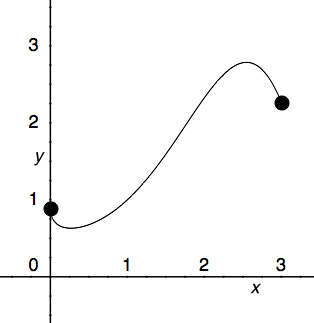#### Question 4 4. What is the approximate instantaneous rate of change at point C?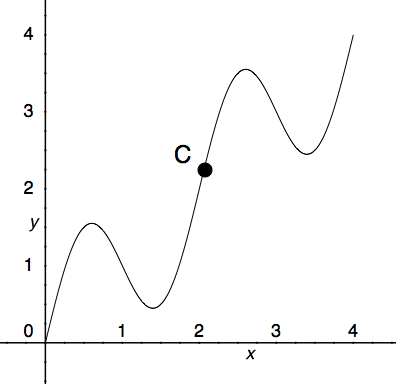#### Question 5 5. Find the derivative df/dt, given the function f(t).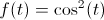### Page 2

#### Question 6 6. Find f'(x) for the following function.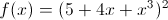#### Question 7 7. Find y'.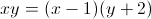#### Question 8 8. Find y'(x).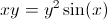#### Question 9 9. Does L'Hopital's rule apply to this problem? Why or why not?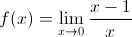#### Question 10 10. According to L'Hopital's rule, which of the following statements MUST be true for the equation shown below to hold? A) f(x) and g(x) approach 0 or infinity B) f'(C) does not equal 0 C) g'(x) does not equal 0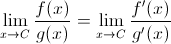### Page 3

#### Question 11 11. Evaluate the derivative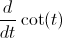#### Question 12 12. Find the derivative of f(x).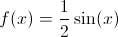#### Question 14 14. In the pictured graph of f(x) = x^2 + 6x, for which values of x is the function increasing?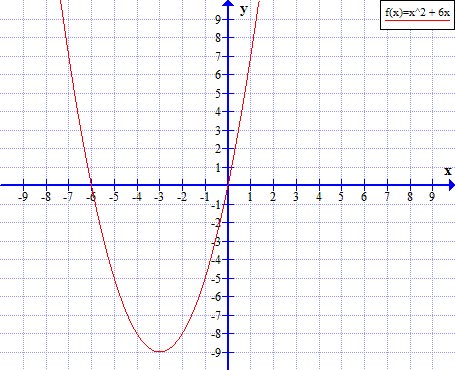#### Question 15 15. This graph represents a(n) _____ function.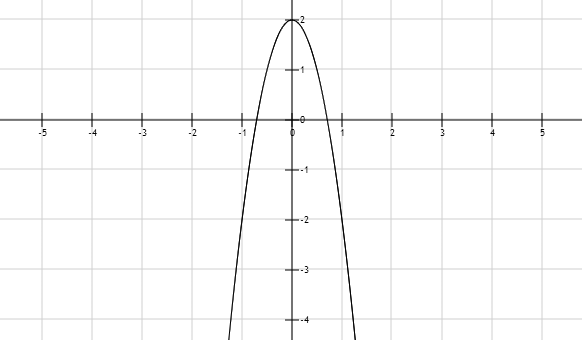### Page 6

#### Question 27 27. Use the given formula to solve for z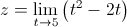#### Question 28 28. Using the given data, solve for z.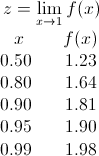#### Differential Calculus & Functions Chapter Exam Instructions

Choose your answers to the questions and click 'Next' to see the next set of questions. You can skip questions if you would like and come back to them later with the yellow "Go To First Skipped Question" button. When you have completed the practice exam, a green submit button will appear. Click it to see your results. Good luck!

Support